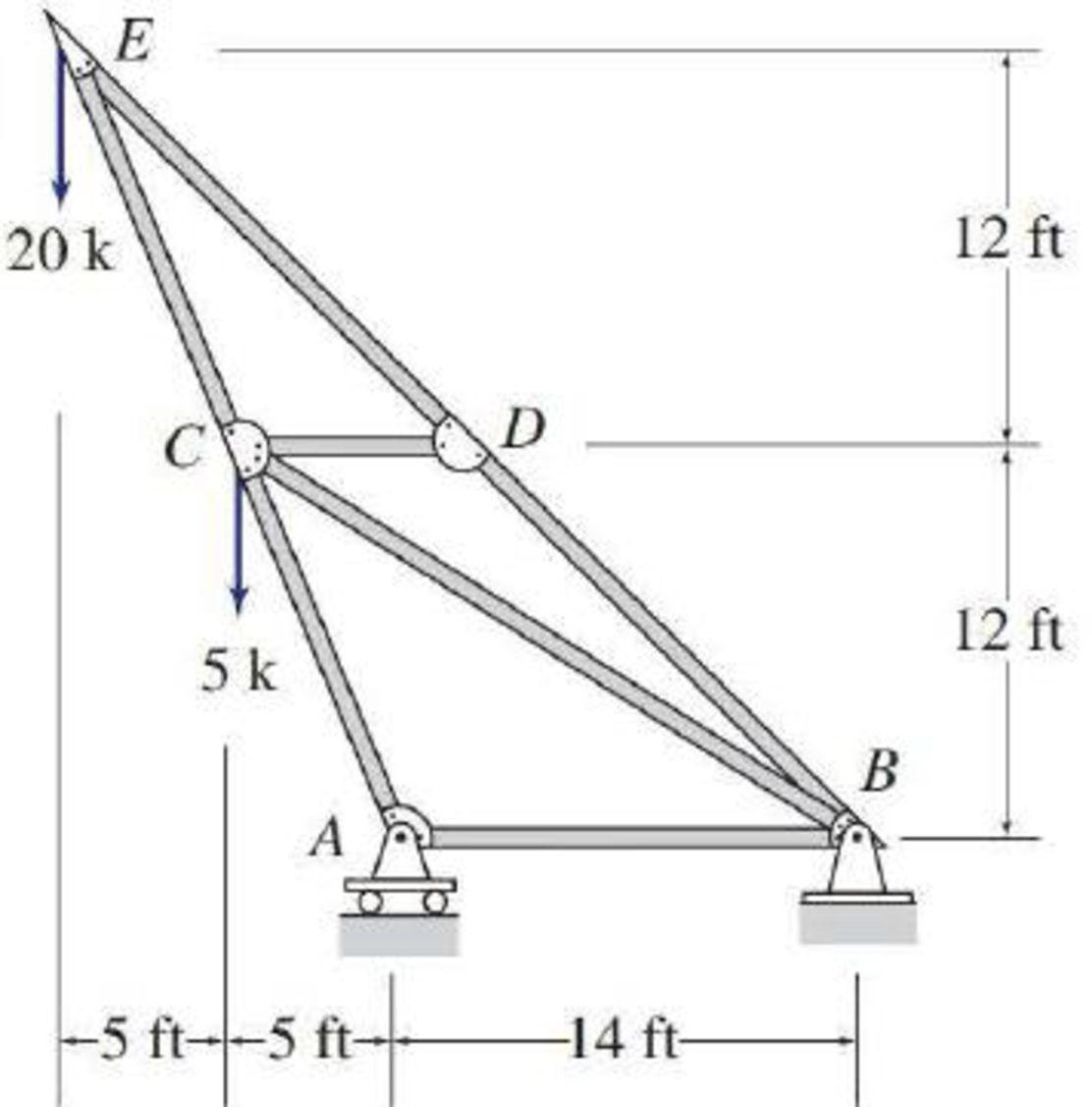# 4.6 through 4.28 Determine the force in each member of the truss shown by the method of joints. FIG. P4.18

#### Solutions

Chapter
Section
Chapter 4, Problem 18P
Textbook Problem
39 views

## 4.6 through 4.28 Determine the force in each member of the truss shown by the method of joints.FIG. P4.18To determine

Find the forces in the members of the truss by the method of joints.

### Explanation of Solution

Given information:

Apply the sign conventions for calculating reactions, forces and moments using the three equations of equilibrium as shown below.

• For summation of forces along x-direction is equal to zero (Fx=0), consider the forces acting towards right side as positive (+) and the forces acting towards left side as negative ().
• For summation of forces along y-direction is equal to zero (Fy=0), consider the upward force as positive (+) and the downward force as negative ().
• For summation of moment about a point is equal to zero (Matapoint=0), consider the clockwise moment as negative and the counter clockwise moment as positive.

Method of joints:

The negative value of force in any member indicates compression (C) and the positive value of force in any member indicates Tension (T).

Calculation:

Consider the forces in the member AC, CE, AB, BD, DE, BC, and CD are FAC,FCE,FAB,FBD,FDE,FBC,FCD.

Show the free body diagram of the truss as shown in Figure 1.

Refer Figure 1.

Consider the vertical reaction at A is Ay.

Consider the horizontal and vertical reactions at B are Bx and By.

Take the sum of the forces in the vertical direction as zero.

Fy=0Ay+By=20+5Ay+By=25        (1)

Take the sum of the forces in the horizontal direction as zero.

Fx=0Bx=0

Take the moment at A and Equate to zero.

MA=014By+5×5+20×10=014By+25+200=0By=22514By=16.07k

Substitute 16.07k for By in Equation (1).

Ay16.07=25Ay=41.07k

Calculate the value of the angles α as follows:

tanα=125α=tan1(125)α=67.38°

Calculate the value of the angles β as follows:

tanβ=1219β=tan1(1219)β=32.27°

Calculate the value of the angles γ as follows:

tanγ=2424γ=tan1(2424)γ=45°

Show the joint A as shown in Figure 2.

Refer Figure 2.

Find the force in the member AC and AB as follows:

Take the sum of the forces in the vertical direction as zero.

Fy=0FACsinα+Ay=0FACsin(67.38°)+41.07=0FAC=[41.07sin(67.38°)]FAC=44.49kFAC=44.49k(C)

Take the sum of the forces in the horizontal direction as zero.

Fx=0FACcosα+FAB=0(44.49)cos(67.38°)+FAB=0FAB=44.49×cos(67.38°)

FAB=17.11kFAB=17.11k(C)

Show the joint B as shown in the Figure 3.

### Still sussing out bartleby?

Check out a sample textbook solution.

See a sample solution

#### The Solution to Your Study Problems

Bartleby provides explanations to thousands of textbook problems written by our experts, many with advanced degrees!

Get Started

Find more solutions based on key concepts
VocabularyState the meaning of the following terms: Physical Law _____ Correlation _____

Engineering Fundamentals: An Introduction to Engineering (MindTap Course List)

Give an example of biometric detection.

Network+ Guide to Networks (MindTap Course List)

What are the main reasons that a mild hybrid consumes less fuel than a conventional vehicle?

Automotive Technology: A Systems Approach (MindTap Course List)

Using Operating System Tools You are the office manager at a social media consulting business. The office recen...

Enhanced Discovering Computers 2017 (Shelly Cashman Series) (MindTap Course List)

What is CAM software?

Precision Machining Technology (MindTap Course List)

What is incident classification?

Management Of Information Security

If your motherboard supports ECC DDR3 memory, can you substitute non-ECC DDR3 memory?

A+ Guide to Hardware (Standalone Book) (MindTap Course List)

What causes most electric shock in the welding industry?

Welding: Principles and Applications (MindTap Course List)